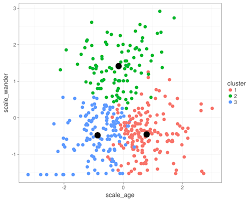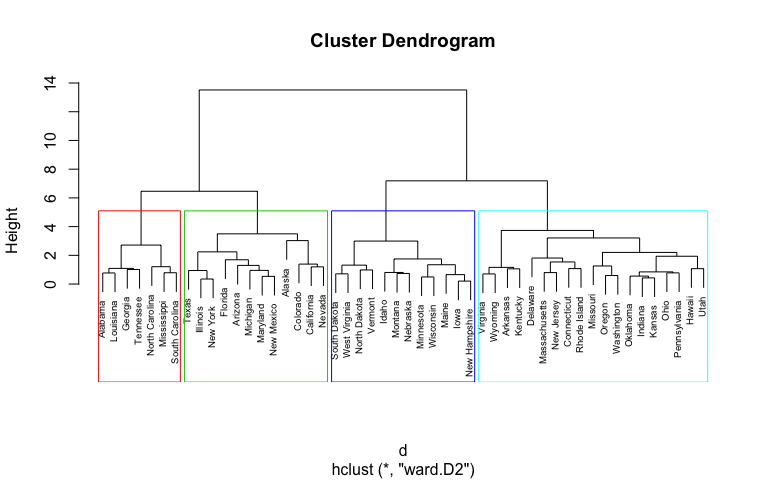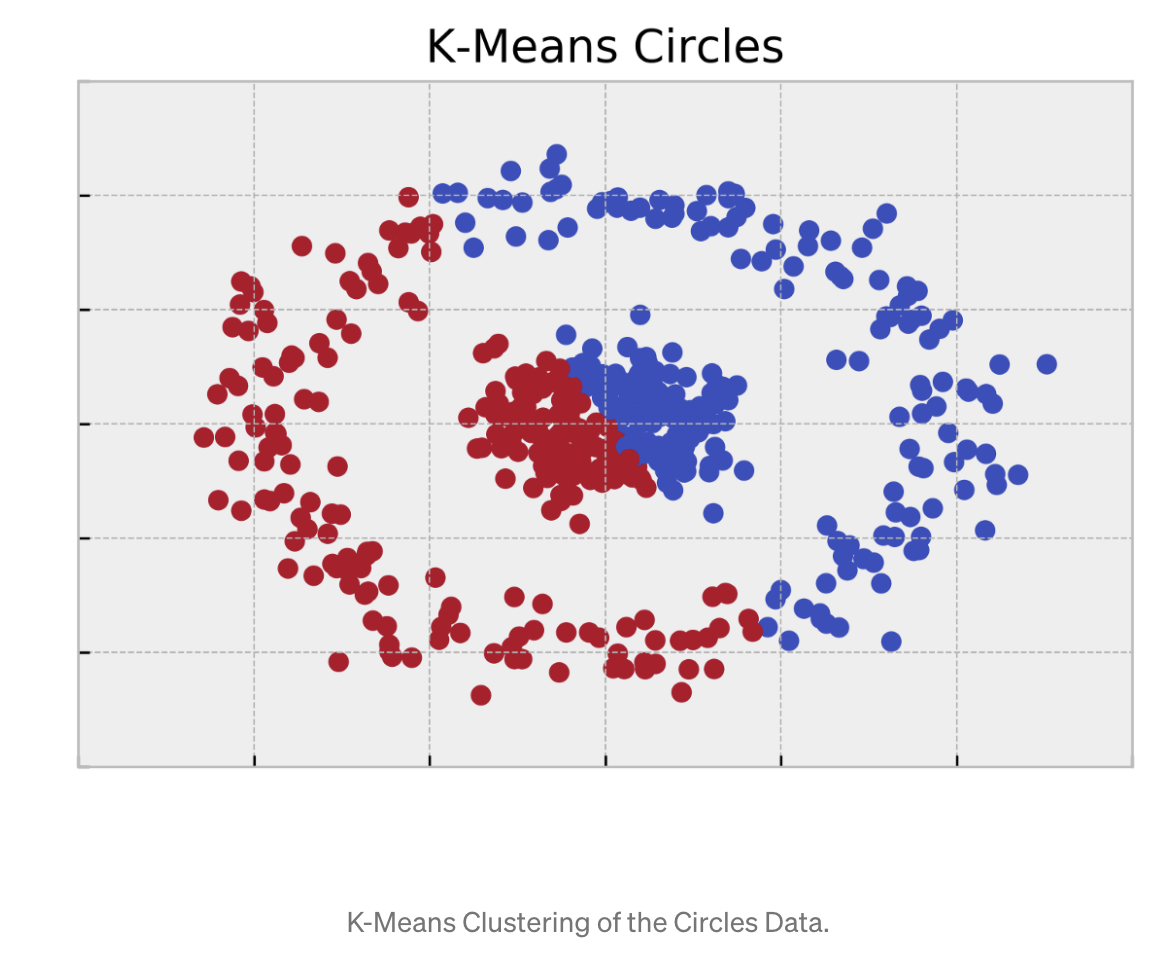# Unsupervised Learning

Based on the “Statistical Consulting Cheatsheet” by Prof. Kris Sankaran

[UNDER CONSTRUCTION]

Essentially, the goal of unsupervised methods is data compression, either to facilitate human interpretation or to improve the quality of downstream analysis. The compressed representation should still contain the signal in present the data, but the noise should be filtered away. Unlike regression and classification (which are supervised), no single variable is of central interest. From another perspective, unsupervised methods can be thought of as inferring latent discrete (clustering) or continuous (factor analysis) “labels” — if they were available, the problem would reduce to a supervised one.

Unsupervised learning encompasses a variety of methods. We go briefly over the following essential directions:

## 1. Clustering

Clustering methods group similar samples with one another. The usual products of a clustering analysis are:

• Cluster assignments: Which samples belong to which clusters?

• Cluster characterization: How can we interpret the resulting clusters?This can be achieved by looking at cluster centroids (the within-cluster averages) or medoids (a sample from within the cluster that is representative of that cluster in some way).

Clustering techniques can roughly be divided up into those that are distance- based and those that are probabilistic.

### 1.1 Distance-based clustering

Distance-based clustering has two main ingredients: (1) a clustering algorithm, (2) a distance/ choice of similarities. Depending on the application, and the problem at hand, these are thus the main “customization” options. In this paragraph, we provide a few classical options. Note however, that you can be creative, adapt and tailor your choices (in particular the choice of the distance) to the problem at hand: the trick usually consists in defining “the right metric”, “the right measure of similarity”, etc.

Step 1: Selecting an Algorithm. Common distance-based methods include:• K-means: This builds K-clusters in an iterative way, attempting to minimize the sum of within-cluster distances (so that the clusters are compact). Since all directions are treated symmetrically, the resulting clusters tend to be spherical. Similarly, since clusters are treated symmetrically, they tend to all be approximately the same size.

• Hierarchical clustering: This builds clusters hierarchically. Specifically, it begins with each sample as its own singleton cluster. The pair that is found to be most similar is then merged together, and the procedure is continued until all clusters are joined into one large cluster. The nearness of a pair of cluster has to be defined somehow: common choices include “complete-link” merging (say that the distance between two clusters is the distance between the furthest away pair across clusters) or “average-link” (say the distance is the average pairwise distance between clusters).An advantage of this approach is that it provides a full tree relating samples. The more branch length a pair of samples shares, the more similar they are. In particular, this approach avoids the problem of choosing K, though clusters can be chosen by cutting the tree at some horizontal level. The main drawback of this approach is that it does not it does not scale as well as fixed-K methods.• Spectral clustering: This method is “spectral”, because it relies on the eigenvector decomposition of the Laplacian of the data: $$L= D - A$$ (where $$A$$ is a similarity matrix between datapoints, and $$D$$ the associated degree) to do a dimensionality reduction of the data before clustering.
The main advantage of this method is that it is non-linear.

•  Kernel k-means: This is a kernelized version of k-means, ie., at a high level, k-means is applied to “high-dimensional embeddings” of the original dataset. This allows to use a richer class of distances, as well as to have non-lnear embeddings.
In these methods, decisions need to be made about (1) preprocessing and (2) the distance to use. Preprocessing will vary on a case-by-case basis. Generally, the goal of preprocessing is to ensure that the features included in clustering are meaningful, which means we might drop, transform (e.g., standardization), or derive features from (e.g., from counts to tf-idf scores) the original raw features.

Step 2: Selecting a distance : As far as distances are concerned, some useful ones to know are:

• Euclidean distance: This is the standard length of a line between two points in a euclidean space: $$d(x_i, x_j) = || x_i - x_j||^2 = \sum_{k=1}^p ( x_{ik} - x_{jk})^2$$. It is a natural default, especially considering it’s connection to squared-error loss / the exponent in the Gaussian. Note that, due to this connection to squared- error, it can be sensitive to outliers.
• Weighted euclidean distance: If we want the coordinates to have different amounts of influence in the resulting clustering, we can reweight the coordinates in the euclidean distance according to $$d(x_i, x_j) = \sum_{k=1}^p w_k ( x_{ik} - x_{jk})^2$$ where $$w_k$$ is the weight associated with coordinate $$k$$. This kind of reweighting can be useful when the relative weights for groups of columns should be made comparable to one another.
• Mahalanobis distance: This is defined as $$d(x_i, x_j) = (x_i-x_j)^T \Sigma^{-1} (x_i -x_j)$$.
Note that ordinary and weighted euclidean distances are special cases, with $$\Sigma = I_p$$ and $$\text{diag}(w)$$, respectively. Geometrically, this distance reweights directions according to the contours defined by the ellipse with eigenvectors of $$\Sigma$$ .
Equivalently, this is the distance that emerges when using an ordinary euclidean distance on the rescaled data set, which premultiplies $$X$$ according to  $$\Sigma^{-1/2}$$.
• Manhattan / $$\ell_1$$-distance: Defined as $$d(x_i, x_j) = \sum_{k=1}^d | x_{ik} - x_{jk}|$$ When applied to binary data, this counts the number of mismatches between coordinates.
• Cosine distance: This measures the size of the angle between a pair of vectors. This can be especially useful when the length of the vectors is irrelevant. For example, if a document is pasted to itself, the word count has doubled, but the relative occurrences of words has remained the same. Formally, this distance is defined as $$d(x_i, x_j) = 1 - \frac{x_i^Tx_j}{||x_i||_2 ||x_j||_2} = 1 - \cos(\theta_{x_i, x_j})$$ where $$\theta_{x_i, x_j}$$ represents the angle between $$x_i$$ and $$x_j$$ .
• Jaccard: This is a distance between pairs of length $$p$$ binary sequences $$x_i$$ and $$x_j$$ defined as $$d(x_i, x_j) = 1 - \frac{ \sum_{k=1}^p 1_{x_{ik} = x_{jk} =1}}{p}$$ or one minus the fraction of coordinates that are 0/1. The motivation for this distance is that coordinates that are both 0 should not contribute to similarity between sequences, especially when they may be dominated by 0s. We apply this distance to the binarized version of the species counts.
• Dynamic time warping distance: A dynamic time warping distance is useful for time series that might not be quite aligned. The idea is to measure the distance between the time series after attempting to align them using dynamic programming.
• Mixtures of distances: Since a convex combination of distances is still a distance, new ones can be tailored to specific problems accordingly. For example, on data with mixed types, a distance can be defined to be a combination of a euclidean distance on the continuous types and a jaccard distance on the binary types.

### 1.2. Probabilistic clustering techniques

In contrast, probabilistic clustering techniques assume latent cluster indicator $$z_i$$ ( e.g $$z_i =k$$ if sample $$i$$ belongs to cluster $$k$$( for each sample and define a likelihood model (which must itself be fit) assuming these indicators are known. Inference of these unknown $$z_i$$’s provides the sample assignments, while the parameters fitted in the likelihood model can be used to characterize the clusters. Some of the most common probabilistic clustering models are:

• Gaussian mixture model: The generative model here supposes that there are $$K$$ means $$\mu_{1; \cdots ; K}$$. Each sample $$i$$ is assigned to one of $$K$$ categories (call it $$z_i = k$$), and then the observed sample is drawn from a gaussian with mean $$\mu_k$$ and covariance $$\Sigma$$  (which doesn’t depend on the class assignment). This model can be considered the probabilistic analog of K-means (K means actually emerges as the small-variance limit).
• Multinomial mixture model: This is identical to the gaussian mixture model, except the likelihood associated with the kth group is $$\text{Mult} (n_i; p_k)$$, where $$n_i$$ is the count in the $$i$$th row. This is useful for clustering count matrices.
• Latent Class Analysis:
• Hidden Markov Model: This is a temporal version of (any of) the earlier mixture models.The idea is that the underlying states zi transition to one another according to a markov model, and the observed data are some emission (gaussian or multinomial, say) from the underlying states. The point is that a sample might be assigned to a centroid different from the closest one, if it would allow the state sequence to be one with high probability under the markov model.

## 2. Low-dimensional representations

• Principle Components Analysis
• Factor analysis
• Distance based methods